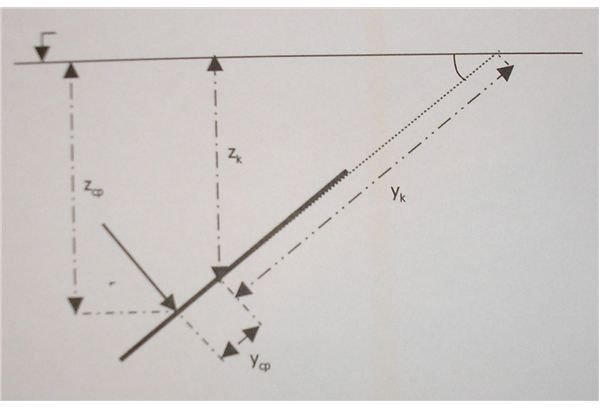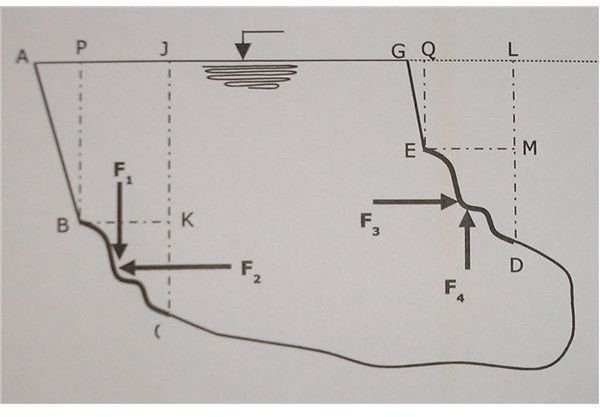# Forces Acting on Plane and Curved Submerged Surfaces

Study of hydrostatic forces on submerged or static surfaces is very important for the design and engineering processes. Construction of dams, installation of underwater hydraulic systems, and forces exerted on ships are some of the important and crucial processes that require study of hydrostatic forces.

## Forces on planar surfaces

If the surface is planar, a single resultant point force is found, mechanically equivalent to the distributed pressure load over the whole surface.

This resultant point force acts compressively, normal to the surface, through a point termed the “center of pressure”.

Its magnitude is: F=γzkA, where:

• γ is the fluid's specific gravity. For water, it is 9810 N/m3.
• zk is the depth in which the center of gravity of the surface, the centroid, is situated.
• A is the surface’s area.

The product (γ.zk), is the hydrostatic pressure at the depth of the centroid of the surface. In case the free surface of the liquid that contains the surface is under atmospheric pressure alone, the above equation is enough to describe the force. But in case the free surface is under additional pressure, this pressure will have an additional effect on the acting force. The value of the pressure in the center of gravity of the surface, is no longer (γ.zk). It is now γ(zk+p/γ), where p is the above-mentioned pressure.

Calculating the magnitude of the force is done as described above. The determination of the point where this force applies, the “center of pressure,” is a little more complicated:

If the surface is inclined at an angle, θ, to the horizontal, the coordinates of the center of pressure, (xcp, ycp), in a coordinate system in the plane of the surface, with origin at the centroid of the surface, are:

xcp = Ixy/(ykA) and ycp = Ixx/(ykA)

where Ixx is the area moment of inertia, Ixy the product of inertia of the plane surface, both with respect to the centroid of the surface, and y is positive in the direction below the centroid.

The surface is often symmetrically loaded, so that Ixx = 0, and hence, xcp = 0, or the center of pressure is located directly below the centroid on the line of symmetry.

If the surface is horizontal, the center of pressure coincides with the centroid. Further, as the surface becomes more deeply submerged, the center of pressure approaches the centroid, that is, (xcp, ycp) approaches to (0,0).## Forces on curved surfaces

For general curved surfaces, it may no longer be possible to determine a single resultant force equivalent to the hydrostatic load; we thus determine separately one or two horizontal components, and a vertical component.

The horizontal component of the force acting on a curved surface is equal with the force that would be acting on a planar surface. This planar surface is the projection of the curved surface on the vertical level. For example in figure 2, where one sees reservoir ABCDEGJPA, the horizontal components with which water pushes surfaces BC and DE, are F2 and F3 respectively. To calculate the magnitude of F2, all we need to do is consider KC, which is the projection of BC on the vertical plane. By determining the force to KC, we have F2. The same holds for F3. It is equal to the force on surface MD.

The vertical component of the force is equal to the weight of the volume of liquid that exists between the surface, and the free surface of the liquid. And this is true whether there is a free surface or not. By this we mean that if the liquid is above the surface, the postulation is true. In this case, the force is directed downwards. In the opposite case, where, the liquid is situated below the surface, the same volume counts. The magnitude of the force is still equal to the weight of the same liquid volume. Only, this volume is now imaginary. The direction of the force in this case is the inverse: upwards.

To express this in another way, if the surface is exposed to the hydrostatic load from above, like the surface BC in Fig. 2, then the force acts downwards. The magnitude of F1 is equal to the weight of the volume PBCJP. If the surface is exposed to a hydrostatic load from below, like the surface DE, then the force acts upwards. And the magnitude of F4 is equal to the weight of the volume QEDLQ of water. It acts through the center of gravity of that fluid volume.

In case a surface is such that there are both upward and downward vertical components, like surface EDC, the net vertical force on the surface is the algebraic sum of upward and downward components.

For general curved surfaces, it may no longer be possible to determine a single resultant force equivalent to the hydrostatic load; we thus determine separately one or two horizontal components, and a vertical component.

The horizontal component of the force acting on a curved surface is equal with the force that would be acting on a planar surface. This planar surface is the projection of the curved surface on the vertical level. For example in figure 2, where one sees reservoir ABCDEGJPA, the horizontal components with which water pushes surfaces BC and DE, are F2 and F3 respectively. To calculate the magnitude of F2, all we need to do is consider KC, which is the projection of BC on the vertical plane. By determining the force to KC, we have F2. The same holds for F3. It is equal to the force on surface MD.

The vertical component of the force acting on a curved surface has a magnitude that is defined as “the weight of the volume of water, or liquid in general, that exists above the surface, and under the free surface.” And this is true, whether there is a free surface, or not. By this we mean that, if the liquid is above the surface, the postulation is true. In this case, the force is directed downwards. In the opposite case, where, the liquid is situated below the surface, the same volume counts. The magnitude of the force is still equal to the weight of the same liquid volume. Only, this volume is now imaginary. The direction of the force in this case is the inverse: upwards.

To express this in another way, if the surface is exposed to the hydrostatic load from above, like the surface BC in Fig. 2, then the force acts downwards. The magnitude of F1 is equal to the weight of the volume PBCJP. If the surface is exposed to a hydrostatic load from below, like the surface DE, then the force acts upwards. And the magnitude of F4 is equal to the weight of the volume QEDLQ of water. It acts through the center of gravity of that fluid volume.

In case a surface is such that there are both upward and downward vertical components, like surface EDC, the net vertical force on the surface is the algebraic sum of upward and downward components.